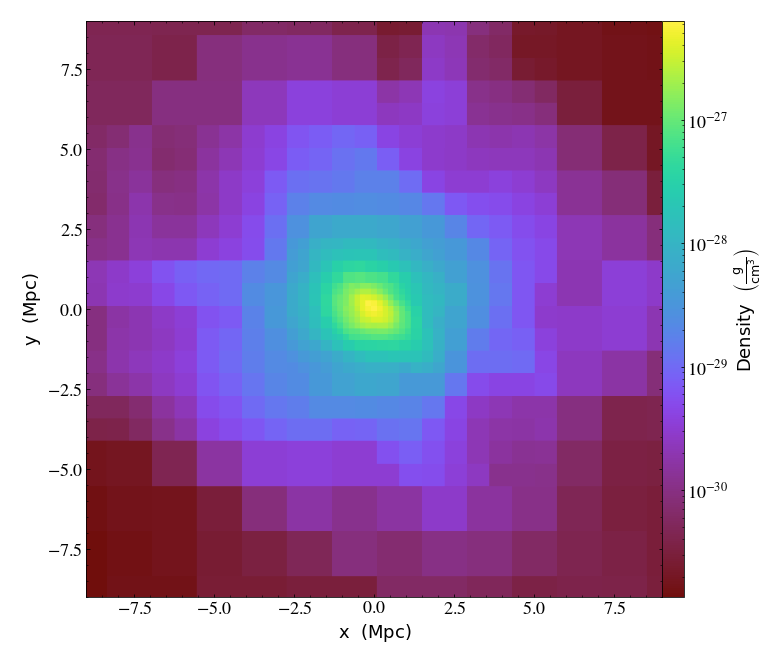Notebook

# Simple Visualizations of Data¶

Just like in our first notebook, we have to load yt and then some data.

In :
import yt


For this notebook, we'll load up a cosmology dataset.

In :
ds = yt.load_sample("enzo_tiny_cosmology")
print ("Redshift =", ds.current_redshift)

Redshift = -2.7810863612032e-09


In the terms that yt uses, a projection is a line integral through the domain. This can either be unweighted (in which case a column density is returned) or weighted, in which case an average value is returned. Projections are, like all other data objects in yt, full-fledged data objects that churn through data and present that to you. However, we also provide a simple method of creating Projections and plotting them in a single step. This is called a Plot Window, here specifically known as a ProjectionPlot. One thing to note is that in yt, we project all the way through the entire domain at a single time. This means that the first call to projecting can be somewhat time consuming, but panning, zooming and plotting are all quite fast.

yt is designed to make it easy to make nice plots and straightforward to modify those plots directly. The cookbook in the documentation includes detailed examples of this.

In :
p = yt.ProjectionPlot(ds, "y", ("gas", "density"))
p.show()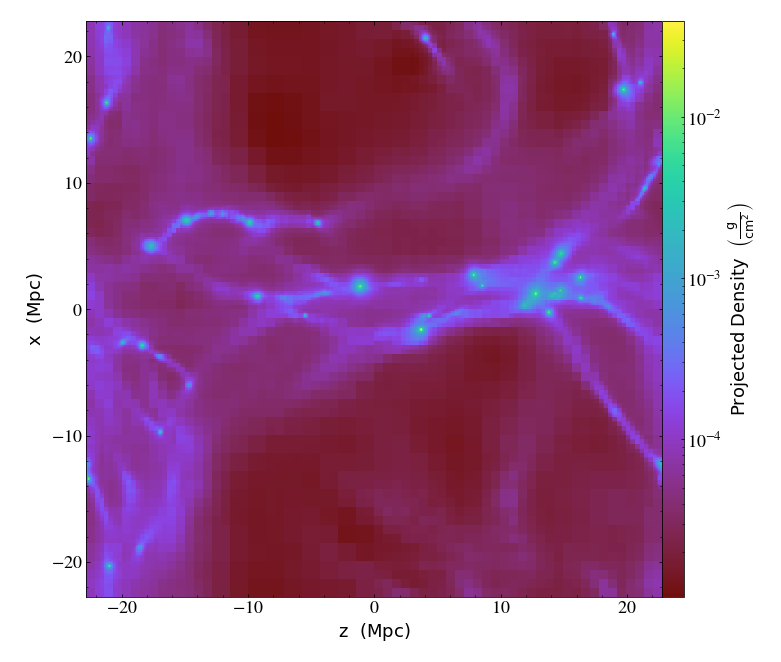The show command simply sends the plot to the IPython notebook. You can also call p.save() which will save the plot to the file system. This function accepts an argument, which will be prepended to the filename and can be used to name it based on the width or to supply a location.

Now we'll zoom and pan a bit.

In :
p.zoom(2.0)

Out: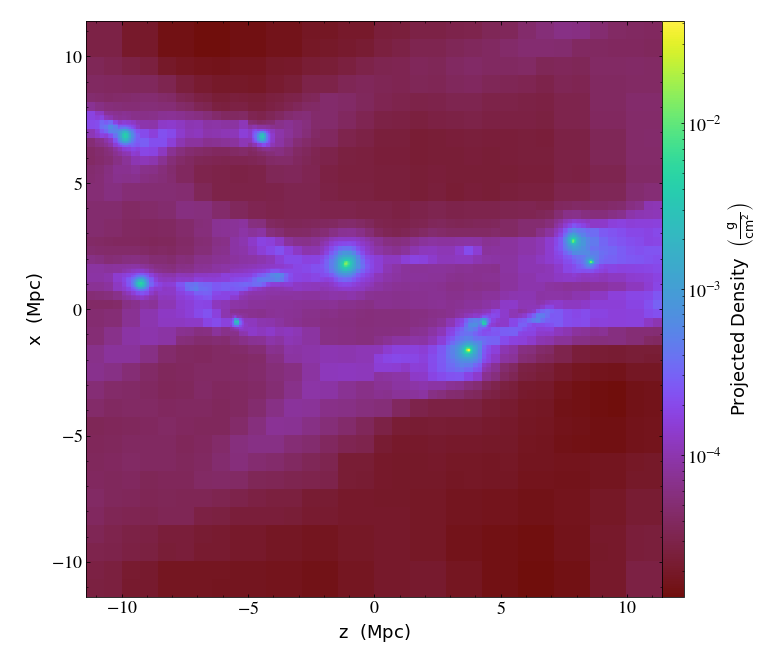In :
p.pan_rel((0.1, 0.0))

Out: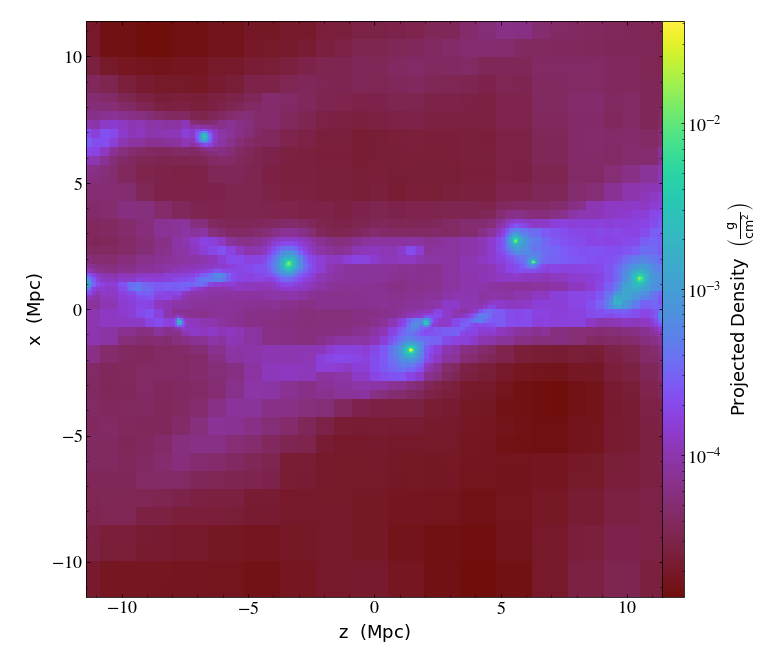In :
p.zoom(10.0)

Out: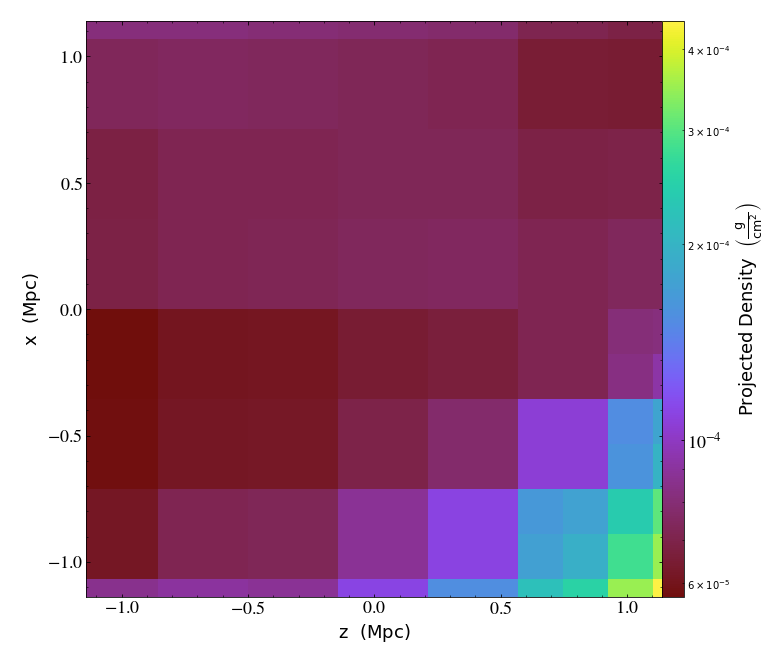In :
p.pan_rel((-0.25, -0.5))

Out: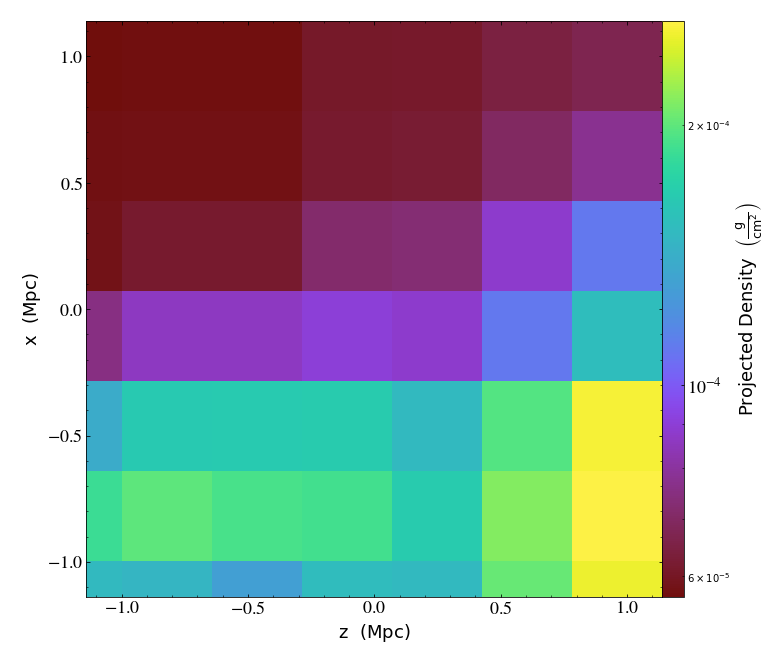In :
p.zoom(0.1)

Out: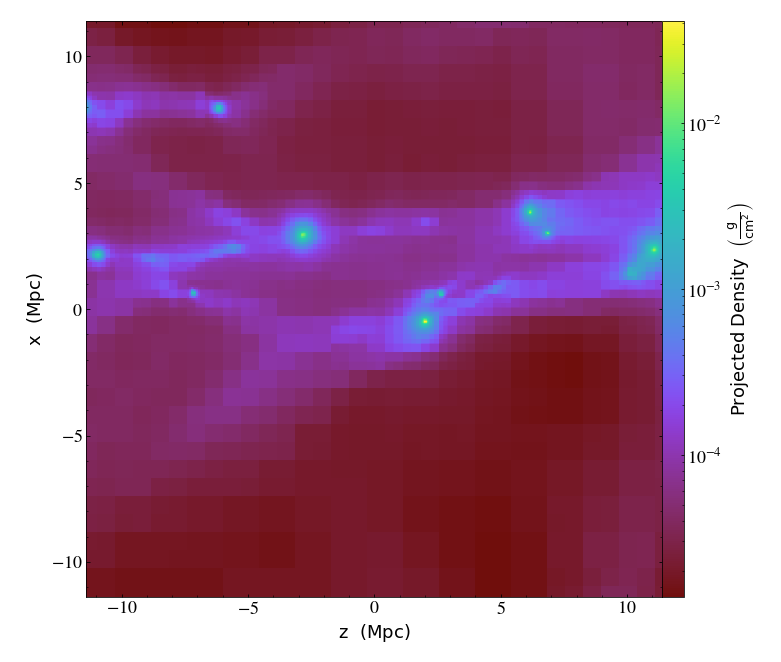If we specify multiple fields, each time we call show we get multiple plots back. Same for save!

In :
p = yt.ProjectionPlot(ds, "z", [("gas", "density"), ("gas", "temperature")], weight_field=("gas", "density"))
p.show()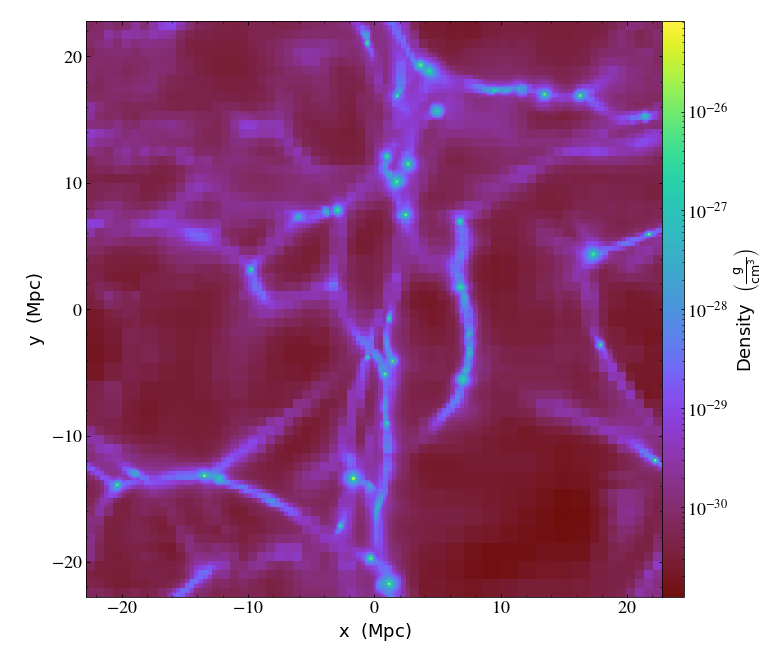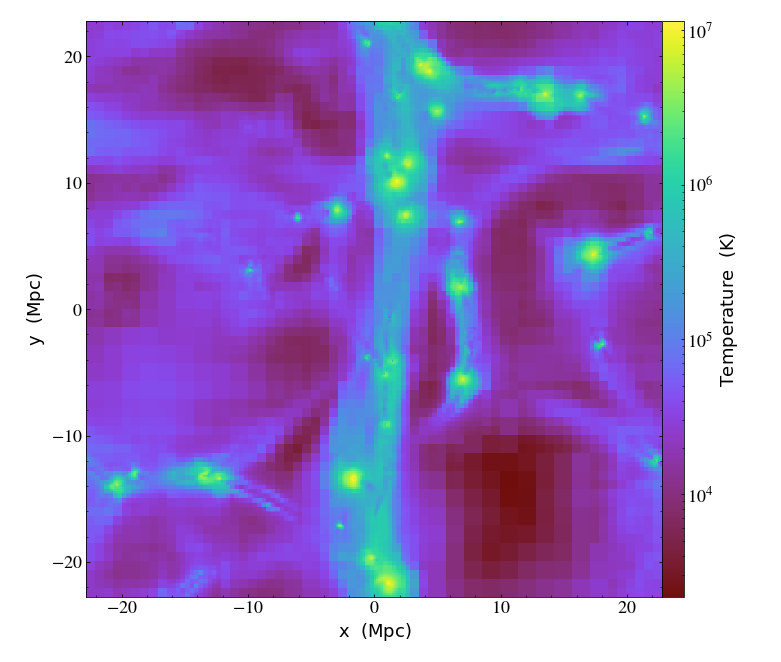We can adjust the colormap on a field-by-field basis.

In :
p.set_cmap(("gas", "temperature"), "hot")

Out: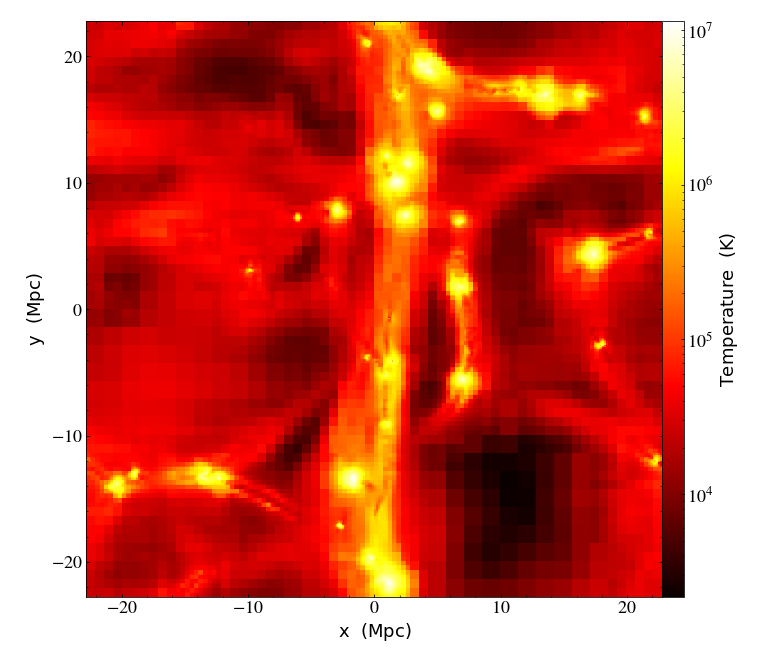And, we can re-center the plot on different locations. One possible use of this would be to make a single ProjectionPlot which you move around to look at different regions in your simulation, saving at each one.

In :
v, c = ds.find_max(("gas", "density"))
p.set_center((c, c))
p.zoom(10)

Out: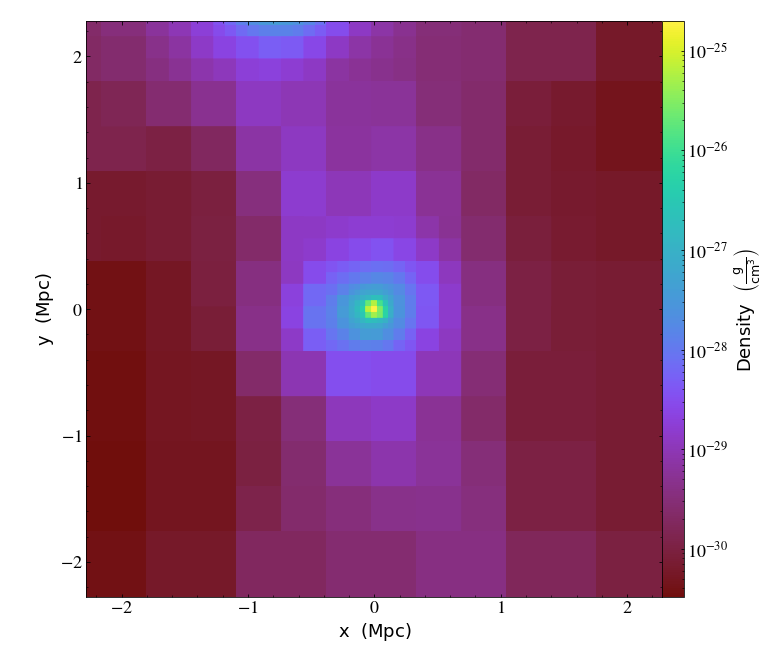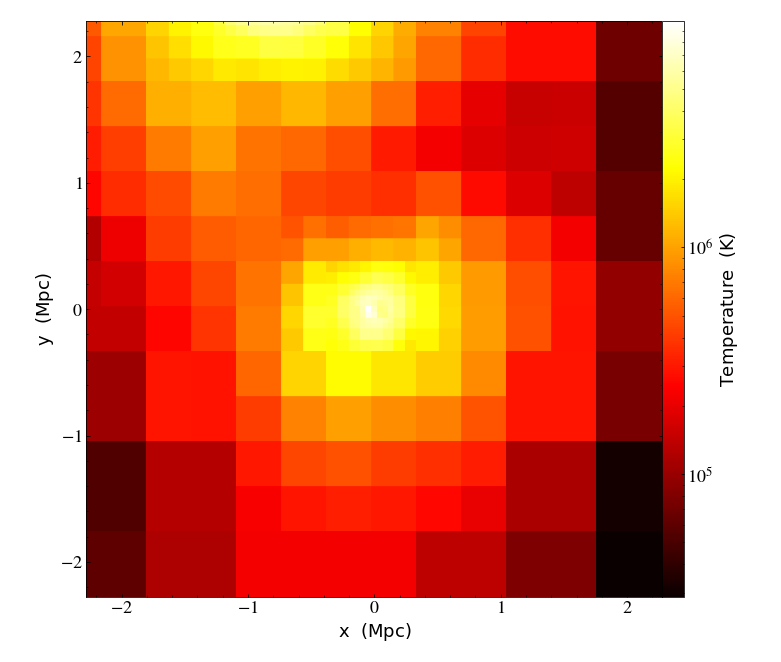Okay, let's load up a bigger simulation (from Enzo_64 this time) and make a slice plot.

In :
ds = yt.load_sample("Enzo_64/DD0043/data0043")
s = yt.SlicePlot(ds, "z", [("gas", "density"), ("gas", "velocity_magnitude")], center="max")
s.set_cmap(("gas", "velocity_magnitude"), "kamae")
s.zoom(10.0)

Out: